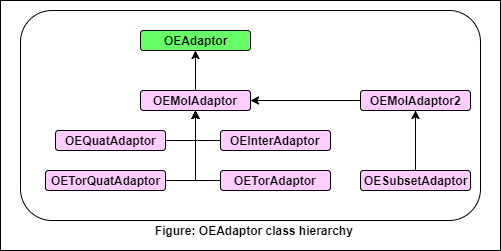# OEAdaptor¶

Attention

This API is currently available in C++ and Python.

class OEAdaptor


The OEAdaptor class is a pure virtual base class which defines the interface for classes which adapt or transform variables and gradients during an optimization. Adaptors are useful in cases where function evaluations are performed in a different coordinate system than the variables being optimized (for example polar coordinates are optimized but the function is evaluated in Cartesian coordinates).The OEAdaptor class defines the following public methods:
The following classes derive from this class:

## AdaptVar¶

bool AdaptVar(double *ca, const double *) const


Takes a set of variables as the second argument and copies a set of transformed variables into the first argument. This method is commonly used to transform optimized variables back into the coordinate system of the original reference variables.

## GetVar¶

void GetVar(double* var, const double* refVar)


Takes reference variables as an argument (refVar), and transforms them into the variable type or coordinate system (var) that will be used during an optimization. Reference variables normally use the same coordinate system as the function evaluations that will be performed in an optimization. Many adaptors take Cartesian coordinates as the argument to the method, and return an array of variables containing the initial adapted state, such as torsion angles or a quaternion.